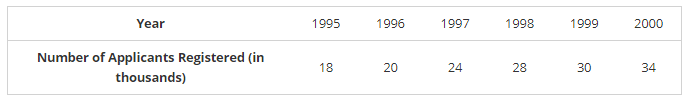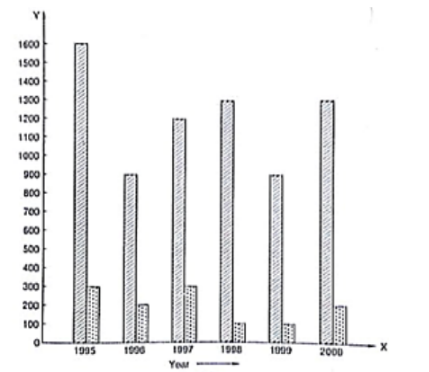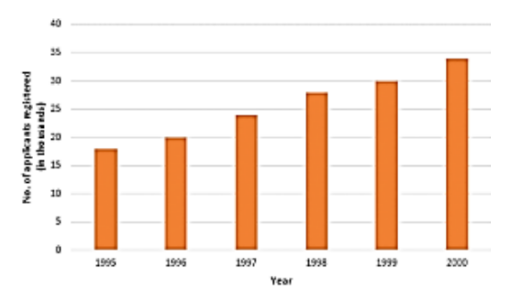# The following data gives the number (in thousands) of applicants registered with an Employment Exchange during 1995 – 2000:

Question:

The following data gives the number (in thousands) of applicants registered with an Employment Exchange during 1995 – 2000:Construct a bar graph to represent the above data.Solution:

To represent the given data by a vertical bar graph, we first draw horizontal and vertical axes. Let us consider that the horizontal and vertical axes represent the years and the number of applicants registered in thousands respectively. We have to draw 6 bars of different lengths given in the table. At first we mark 6 points in the horizontal axis at equal distances and erect rectangles of the same width at these points. The heights of the rectangles are proportional to the number of applicants registered.

The vertical bar graph of the given data is following: